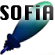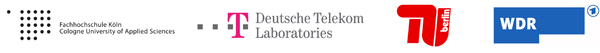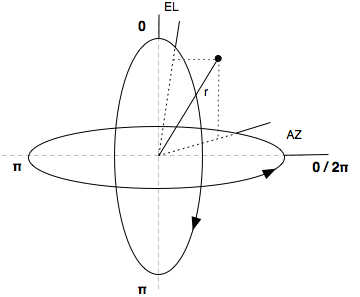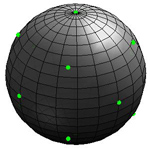Welcome to SOFiA
Who is behind SOFiA
Feature overview
System overview
Function reference
mergeArrayData
F/D/T
gauss
lebedev
S/W/G
S/T/C
W/G/C
S/F/E
M/F
R/F/I
P/D/C
I/T/C
makeMTX
makeIR
visual3D
Coordinate System
Application Examples
Example 1
Example 2
Example 3
Example 4
Example 5
Example 6
Example 7
Example 8
Array Datasets
VariSphear system
Groups and Mailinglists
Contact and Support
How to Reference

# SOFiA Coordinate System

SOFiA is based on a spherical coordinate system as follows:
AZ denotes the azimutal angle in a range of (0-2pi(. Whereas AZ=0 is defined to be the front direction and AZ=pi to be the rear direction.
EL denotes the elevation angle in a range of (0-pi). EL=0 points upwards, EL=pi/2 points to the horizontal plane and EL=pi points downwards.
All angles are in RAD.
r is the radius in meters (if needed/specified).## EXAMPLE

14-Point Lebedev GridAZ EL
0.0000
0.0000
0.0000
1.5708
0.0000
3.1416
0.7854
2.1863
0.7854
0.9553
1.5708
1.5708
2.3562
2.1863
2.3562
0.9553
3.1416
1.5708
3.9270
2.1863
3.9270
0.9553
4.7124
1.5708
5.4978
2.1863
5.4978
0.9553